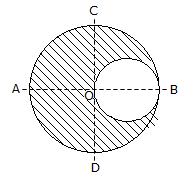Last updated: August 14, 2020

1. The angle of the inclined plane at which a body just begins to slide down the plane, is called helix angle.
2. A circular hole of 50 mm diameter is cut out from a circular disc of 100 mm diameter as shown in the below figure. The centre of gravity of the section will lie3. The maximum velocity of a particle moving with simple harmonic motion is
4. Moment of inertia of a rectangular section having width (b) and depth (d) about an axis passing through its C.G. and parallel to the width (b), is
5. The resultant of two equal forces P making an angle θ, is given by
6. The velocity ratio for the first system of pulleys is(where n is the number of pulleys.)
7. 1 joule means that
8. The ideal angle of banking provided on the curves on roads depends upon
9. The linear velocity of a body rotating at ω rad / s along a circular path of radius r is given by
10. A non-reversible machine is also called a self-locking machine.
Engineering Mechanics MCQ 10
You got {{userScore}} out of {{maxScore}} correct
{{title}}
{{image}}
{{content}}